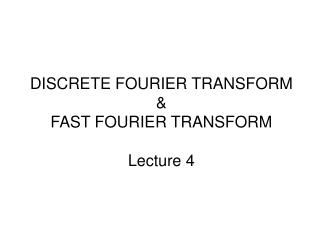# DISCRETE FOURIER TRANSFORM & FAST FOURIER TRANSFORM Lecture 4 - PowerPoint PPT PresentationDownload PresentationDISCRETE FOURIER TRANSFORM & FAST FOURIER TRANSFORM Lecture 4

DISCRETE FOURIER TRANSFORM & FAST FOURIER TRANSFORM Lecture 4Download Presentation## DISCRETE FOURIER TRANSFORM & FAST FOURIER TRANSFORM Lecture 4

- - - - - - - - - - - - - - - - - - - - - - - - - - - E N D - - - - - - - - - - - - - - - - - - - - - - - - - - -
##### Presentation Transcript

1. DISCRETE FOURIER TRANSFORM & FAST FOURIER TRANSFORM Lecture 4

2. Decimation-in-Frequency FFT Algorithm x(0), x(1), … , x(N-1) let m = n-N/2 (n = N/2+m) n = N/2 => m = N/2-N/2 = 0n = N-1 => m = N-1-N/2 = N/2-1

3. X(k) : N-point DFT of x(0), …, x(N)  two N/2 point DFT